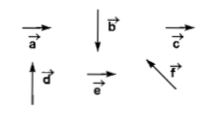Six vector  have the directions indicated in the figure.Which of the following statements may be true?(a) $\stackrel{\to }{b}+\stackrel{\to }{c}=\stackrel{\to }{-f}$                        (b) $\stackrel{\to }{d}+\stackrel{\to }{c}=\stackrel{\to }{f}$

(c) $\stackrel{\to }{d}+\stackrel{\to }{e}=\stackrel{\to }{f}$                        (d) $\stackrel{\to }{b}+\stackrel{\to }{e}=\stackrel{\to }{f}$